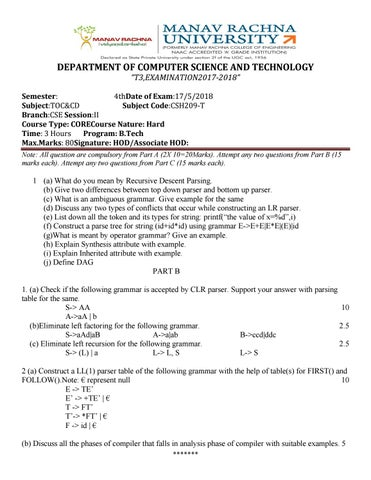DEPARTMENT OF COMPUTER SCIENCE AND TECHNOLOGY “T3,EXAMINATION2017-2018” Semester: 4thDate of Exam:17/5/2018 Subject:TOC&CD Subject Code:CSH209-T Branch:CSE Session:II Course Type: CORECourse Nature: Hard Time: 3 Hours Program: B.Tech Max.Marks: 80Signature: HOD/Associate HOD: Note: All question are compulsory from Part A (2X 10=20Marks). Attempt any two questions from Part B (15 marks each). Attempt any two questions from Part C (15 marks each).

1 (a) What do you mean by Recursive Descent Parsing. (b) Give two differences between top down parser and bottom up parser. (c) What is an ambiguous grammar. Give example for the same (d) Discuss any two types of conflicts that occur while constructing an LR parser. (e) List down all the token and its types for string: printf(“the value of x=%d”,i) (f) Construct a parse tree for string (id+id*id) using grammar E->E+E|E*E|(E)|id (g)What is meant by operator grammar? Give an example. (h) Explain Synthesis attribute with example. (i) Explain Inherited attribute with example. (j) Define DAG PART B 1. (a) Check if the following grammar is accepted by CLR parser. Support your answer with parsing table for the same. S-> AA 10 A->aA | b (b)Eliminate left factoring for the following grammar. 2.5 S->aAd|aB A->a|ab B->ccd|ddc (c) Eliminate left recursion for the following grammar. 2.5 S-> (L) | a L-> L, S L-> S 2 (a) Construct a LL(1) parser table of the following grammar with the help of table(s) for FIRST() and FOLLOW().Note: € represent null 10 E -> TE’ E’ -> +TE’ | € T -> FT’ T’-> *FT’ | € F -> id | € (b) Discuss all the phases of compiler that falls in analysis phase of compiler with suitable examples. 5 *******

3(a) Construct a LR(0) parser for the following grammar and highlight the conflicts (if any) in the table. S->Aa | bAc | dc | bda 10 A-> d 3(b) Give two differences between compiler and interpreter. Discuss all the phases of compiler that falls in synthesis phase of compiler with examples. 2+3 PART C 1.(a) Discuss following methods of code optimization along with suitable examples. 5*2=10 Strength Reduction, Dead Code Elimination, Constant Folding, Redundancy Elimination, Simplification (b) Construct an SDT for input string: 2*3+4 and determine the output for the same. S-> ER R-> *E{printf(“*”);}R |R E-> F+ E { printf(“+”);} |F F-> num {printf(num.val);}

5

2. (a) Represent input string (a*b)+(a*b*c)+(d/e*f) with the help of syntax tree, directed acyclic graph, postfix notation and three address code. 4*2=8 (b) What do you understand by the term three address code? Also using (a*b)+(a*b*c)+(d/e*f) as string construct the tables for Quadruples and Triples 1+2*3=7 3. (a) Discuss three methods of loop optimization. (b) Write three address code for the following expression If a<b && c>d then t=1 else e=0

3*2=6 4

(c) Explain the concept of tokenization. Generate three address code from the following DAG where (-) represent minus sign and (+) represent addition sign 3+2

*******